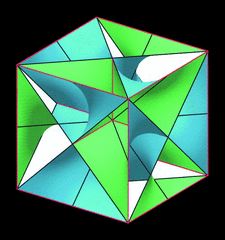issue contentsFOUNDATIONSADVANCES
ISSN: 2053-2733

January 2000 issueCover illustration: Unit cell of a self-intersecting minimal surface with symmetry I432. The surface subdivides R3 into two congruent three-periodic mutually interpenetrating labyrinths with symmetry P4232. Lines of self-intersection are marked in red. See Koch [Acta Cryst. (2000), A56, 15-23].

editorial

Acta Cryst. (2000). A56, 1
doi: 10.1107/S0108767399015871

New developments for Acta Crystallographica Section A

Editorial for 2000.

research papers

Acta Cryst. (2000). A56, 2-10
doi: 10.1107/S0108767399010211

Resonant X-ray scattering in the presence of several anisotropic factors

The general form of the resonant part of the X-ray susceptibility tensor near absorption edges is found when there are several anisotropic factors in a crystal. The simultaneous existence of anisotropy of local atomic environment, magnetic ordering and orbital ordering is considered in detail.

Acta Cryst. (2000). A56, 11-14
doi: 10.1107/S0108767399010478

Diffraction analysis of decorated Fibonacci chains in the average unit-cell approach

A novel approach to diffraction analysis of decorated quasicrystals is discussed. An average unit cell has been constructed for a decorated Fibonacci chain and used for analysis of its diffraction pattern.

Acta Cryst. (2000). A56, 15-23
doi: 10.1107/S0108767399009137

Minimal surfaces with self-intersections along straight lines. II. Surfaces forming three-periodic labyrinths

Minimal surfaces with straight self-intersections may subdivide R3 into two, four or eight three-periodic labyrinths. The properties of such surfaces (three orientable and 44 non-orientable ones) are described.

Acta Cryst. (2000). A56, 24-28
doi: 10.1107/S0108767399011009

Atoms in crystals – from experimental charge densities

The atoms in crystals partitioning scheme of the electron density has been implemented in the program VALRAY and some examples of integrated atomic properties are given using two recent charge-density studies.

Acta Cryst. (2000). A56, 29-35
doi: 10.1107/S0108767399009605

Structures of nanometre-size crystals determined from selected-area electron diffraction data

The structures of a new modification of Ti2Se and several related metal-rich compounds were solved from selected-area electron diffraction data using quasi-automatic direct methods.

Acta Cryst. (2000). A56, 36-48
doi: 10.1107/S010876739901048X

The arithmetic symmetry of monoatomic 2-nets

It is well known that there exist five arithmetic (Bravais) types of 1-nets (two-dimensional simple lattices). We show that there exist also five distinct arithmetic types of monoatomic 2-nets (two-dimensional lattices with two identical points on their unit cell).

Acta Cryst. (2000). A56, 49-61
doi: 10.1107/S0108767399010533

Icosahedral quasiperiodic packing of fibres parallel to fivefold and threefold axes

Building rules are determined for icosahedral quasiperiodic packings of fibres of circular cross section whose axes are parallel to either threefold icosahedral axes or both fivefold and threefold icosahedral axes.

Acta Cryst. (2000). A56, 62-67
doi: 10.1107/S0108767399010818

Prospects for kinematical least-squares refinement in polymer electron crystallography

Electron diffraction intensities from poly(1-butene) are the basis for least-squares structure refinement. With soft constraints placed on bond lengths, an improved structural model can be obtained, despite multiple-scattering perturbations.

Acta Cryst. (2000). A56, 68-72
doi: 10.1107/S0108767399011174

Distribution and interference functions for two-dimensional hexagonal paracrystals

The distribution and scattering interference functions are calculated for two-dimensional hexagonal paracrystals. This modelling respects the hexagonal symmetry, which is not the case for general modelling.

Acta Cryst. (2000). A56, 73-84
doi: 10.1107/S0108767399011277

Fixed-scale wavelet-type approximation of periodic density distributions

Mathematical tools are developed for the decomposition of a distribution in the unit cell into a weighted sum of shifted copies of the same function chosen in advance.

Acta Cryst. (2000). A56, 85-91
doi: 10.1107/S0108767399005838

Microdomain model analysis on the negative partial intensity in a disordered ternary alloy

Short-range ordering in a ternary alloy is discussed according to the ordered microdomain model. The negative X-ray partial intensity can be explained by a mixing occupation of different atoms on the sublattice of the ordered lattice.

Acta Cryst. (2000). A56, 92-95
doi: 10.1107/S0108767399012830

Double crystals

An explanation and proof are given of the different kinds of cost-minimizing planar double crystals that can occur, assuming an interface that carries a fraction of the energy (per unit length) of the faces.

Acta Cryst. (2000). A56, 96-98
doi: 10.1107/S0108767399012283

Symmetry Elements in Space Groups and Point Groups. Addenda to two IUCr Reports on the Nomenclature of Symmetry

An ambiguity in the definition of symmetry element' is removed by redefining the geometric element'. A revised diagram of glide-plane aspects is presented that contains three more than the 15 illustrated previously.

international union of crystallography

Notes for Authors

Follow Acta Cryst. A